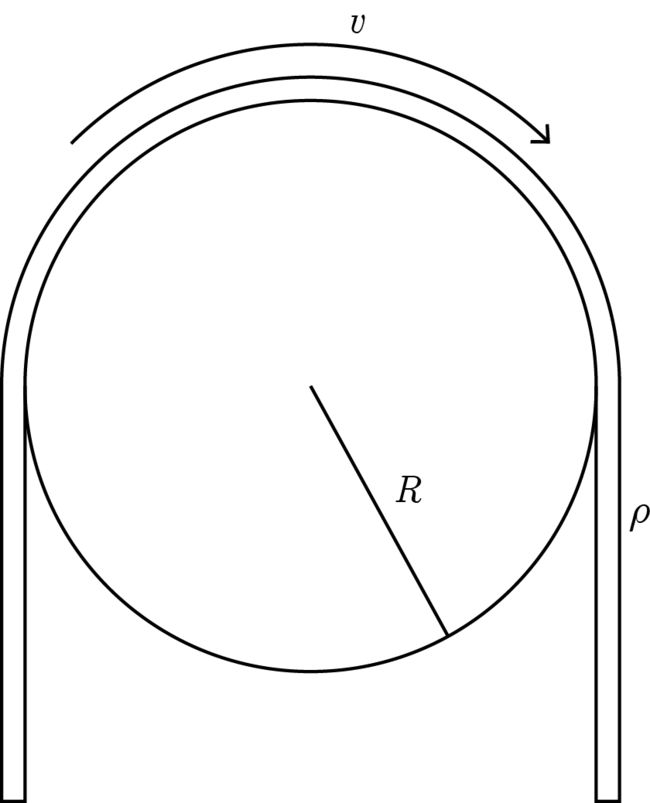# All that matters is the constant velocity!There is a thin string of linear mass density $\rho$, which is going around a pulley of radius $R$, with constant velocity $v$.

Determine the internal tension in the string.

Details and Assumptions

• There is no net force acting on the pulley-string system.

• Neglect gravity.

• Take $\rho = 0.25 \text{ kgm}^{-1}, R = 0.25 \text{ m}, v = \sqrt{13} \text{ ms}^{-1}$.

×

Problem Loading...

Note Loading...

Set Loading...